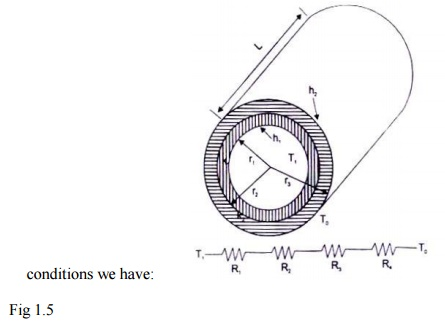Home | | Heat and Mass Transfer | One Dimensional Steady State Heat conduction Composite Systems

# One Dimensional Steady State Heat conduction Composite Systems

There are many practical situations where different materials are placed m layers to form composite surfaces, such as the wall of a building, cylindrical pipes or spherical shells having different layers of insulation.

ONE DIMENSIONAL STEADY STATE HEATCONDUCTION COMPOSITE SYSTEMS:

Composite Surfaces

There are many practical situations where different materials are placed m layers to form composite surfaces, such as the wall of a building, cylindrical pipes or spherical shells having different layers of insulation. Composite surfaces may involve any number of series and parallel thermal circuits.

Heat Transfer Rate through a Composite Wall

Let us consider a general case of a composite wall as shown m Fig. 1.5 The different materials of thicknesses L1, L2, etc and having thermal conductivities kl, k2, etc. On one side of

the composite wall, there is a fluid A at temperature TA and on the other side of the wall there is a fluid B at temperature TB. The convective heat transfer coefficients on the two sides of the wall are hA and hB respectively. The system is analogous to a series of resistances as shown in the figure.Fig 1.4   Heat transfer through a composite wall

The Equivalent Thermal Conductivity

The process of heat transfer through compos lie and plane walls can be more conveniently compared by introducing the concept of 'equivalent thermal conductivity', keq. It is defined as:And,  its  value  depends  on  the  thermal  and  physical  properties  and  the  thickness  of  each constituent of the composite structure.

An Expression for the Heat Transfer Rate through a Composite Cylindrical System

Let us consider a composite cylindrical system consisting of two coaxial cylinders, radii r1, r2 and r2 and r3, thermal conductivities kl and k2 the convective heat transfer coefficients at the inside andoutside surfaces h1 and h2 as shown in the figure. Assuming radial conduction under steady stateconditions we have: Fig 1.5

R1 =1/ h1A1   =1/ 2  1 pLh1

R2 =ln (r2 / r1 )2 pLk1

R3 =ln (r3 / r2 )2 pLk2Study Material, Lecturing Notes, Assignment, Reference, Wiki description explanation, brief detail
Mechanical : Heat and Mass Transfer : Conduction : One Dimensional Steady State Heat conduction Composite Systems |